you are here:/ News & Insights / Engineering Advantage Blog / Determining Compressibility Properties for Hyperelastic FEA Models

# Determining Compressibility Properties for Hyperelastic FEA ModelsDecember 19, 2014 By: Eric Stamper

How do you determine the compressibility of a material and then use that information in a finite element simulation? Answer: by physically testing it and fitting the data to an available material model. In the past, I've sent out rubber material samples to be tested under uniaxial, biaxial, shear and volumetric conditions to obtain the test data that's necessary to generate the coefficients for a hyperelastic material model. There is an abundance of information available on how to fit this type of test data to a Mooney-Rivlin or Odgen model, to name a couple; however there seems to be less information available related to the compressibility aspect, likely because it's common to assume in these models that they're incompressible (i.e. the Poisson's ratio is close to 0.5 or the bulk modulus approaches infinity). If you don't want to assume that the material is completely incompressible, a volumetric test is needed, which leads to this blog topic!

A Pressure-Volume-Temperature (PVT) test can be performed to obtain material compressibility data. This test characterizes the compressibility and volumetric expansion of the material by measuring the change in volume of the test sample due to a change in pressure (or temperature). This data is output in the form of volumetric strain and hydrostatic stress just like what is shown in the table below and if this data was plotted, the slope of the curve would be the bulk modulus (hydrostatic stress = - bulk modulus * volumetric strain). When this data is input into a finite element code, e.g. ANSYS, it might be required that the volumetric strain is in terms of a volume ratio which is what's shown in the graph of the tabular data (volumetric strain = 1 - volume ratio "J").

Depending upon the material model that is fit to this data, the incompressibility can be represented as a linear slope (i.e Mooney-Rivlin) or a higher order fit (i.e. Odgen), etc.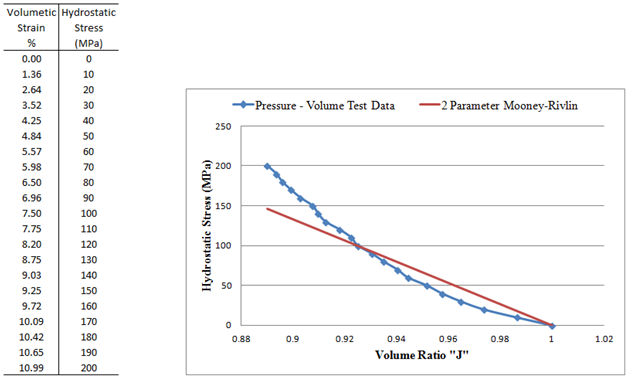Looking at just the volumetric aspect of all the different Mooney-Rivlin models, they all have a single incompressibility constant "d" in the volumetric term of the strain-energy potential (as shown for the 2 parameter Mooney-Rivlin):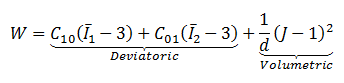Where: d = incompressibility parameter, J = volume ratio (deformed volume over the undeformed volume).

If a straight line is fit through the test data as shown in the above plot for the "2 Parameter Mooney-Rivlin" line, the incompressibility parameter "d" can be calculated from the following relationship to the bulk modulus "K":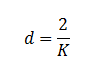Therefore; 2 divided by the slope of this line gives the incompressibility parameter "d". Note that a regression analysis could be used to calculate the line by weighting all data points or treating them equally. In this plot, the secant slope of each data point was calculated first and then averaged to calculate the fit.

There are other codes and material models, LS-DYNA for example, that use the Poisson's ratio "ν" in place of the incompressibility parameter "d". In such cases (e.g. *MAT_HYPERELASTIC_RUBBER), the Poisson's ratio can be calculated once all the material constants are known (in this case: C10, C01 and d).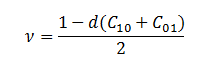The Poisson's ratio came out to 0.49985 for this material, which verified with test data that the assumption of "hyperelastic materials are generally treated as incompressible" was appropriate in this case.

To expand a little more on the example shown, if a higher order fit was desired for the incompressibility parameter "d", the Ogden model could be used which has the following volumetric representation of the strain-energy potential: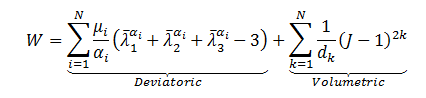The Ogden model can be converted to the same "2 parameter Mooney-Rivlin model" by setting N=2, α1= 2, α2= -2, however; the volumetric component will contain two incompressibility terms: d1 and d2.

Using the ANSYS curve fitting routines this time to perform the regression analysis and determine the values for d1 and d2, the incompressibility fit matches the test data more accurately.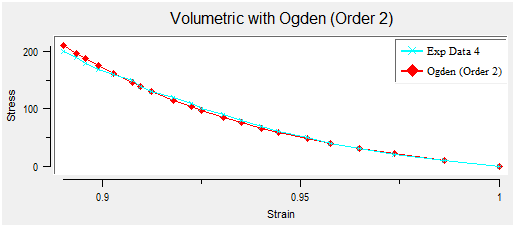Reference: Equations shown in this blog were obtained from the ANSYS theory manual.